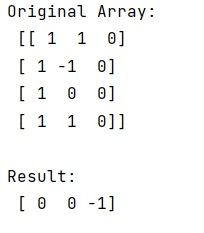# How to find first non-zero value in every column of a NumPy array?

Learn, how to find first non-zero value in every column of a NumPy array in Python?
Submitted by Pranit Sharma, on February 22, 2023

NumPy is an abbreviated form of Numerical Python. It is used for different types of scientific operations in python. Numpy is a vast library in python which is used for almost every kind of scientific or mathematical operation. It is itself an array which is a collection of various methods and functions for processing the arrays.

## Finding the first non-zero value in every column of a NumPy array

Suppose that we are given a numpy array and we need to find the indices of the first index (for every column) where the value is non-zero.

For this purpose, we will define a function inside which we can use numpy.argmax() along that axis (zeroth axis for columns here) on the mask of non-zeros to get the indices of first matches.

Let us understand with the help of an example,

## Python code to find first non-zero value in every column of a NumPy array

```# Import numpy
import numpy as np

# Creating a numpy array
arr = np.array([[1,1,0],[1,-1,0],[1,0,0],[1,1,0]])

# Display original array
print("Original Array:\n",arr,"\n")

# Defining a function
def fun(arr, axis, invalid_val=-1):

# Calling the function
res = fun(arr, 0)

# Display result
print("Result:\n",res,"\n")
```

Output: